# 本文结构思维导图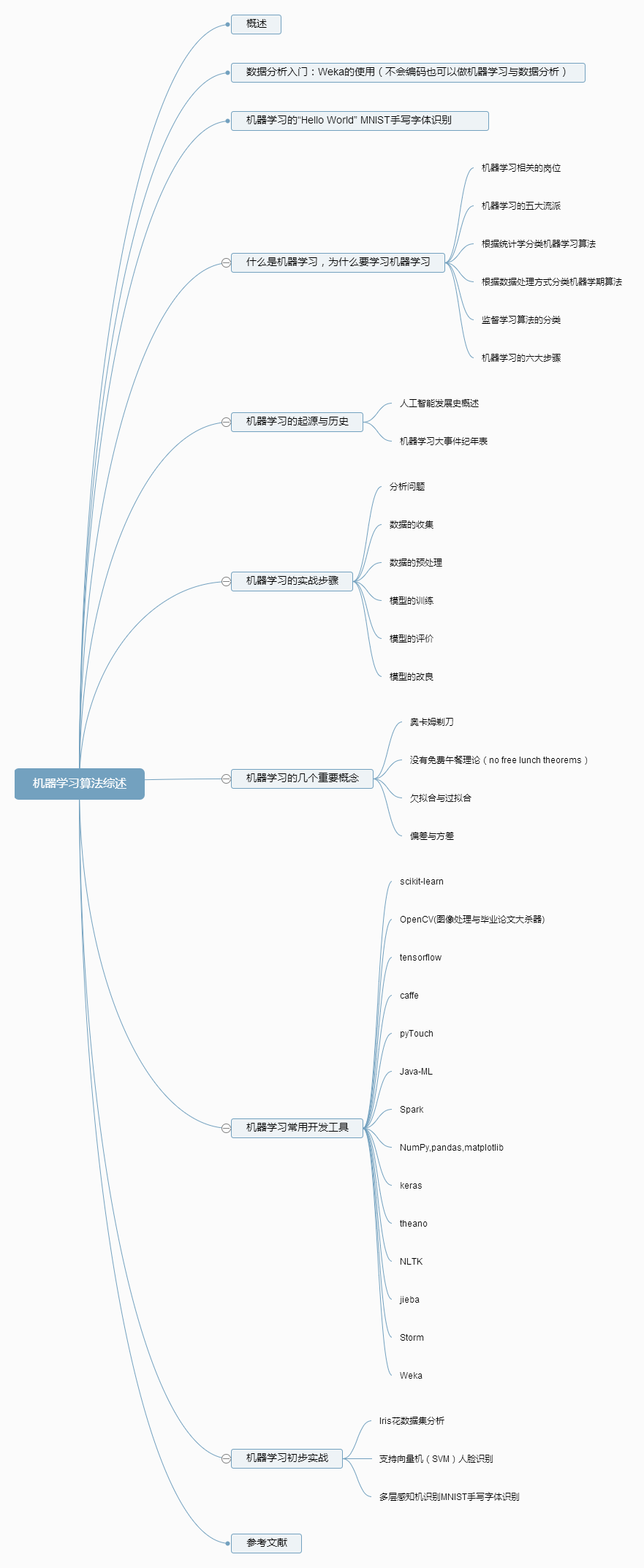# 概述

1. 对于本文中数据集，训练集，特征点，空间等基础概念，请参考周志华老师的《机器学习》第一章的内容
2. Python的语法并不难理解，有编码基础的可以直接看懂，如果不懂，请参考廖雪峰老师的个人博客中Python的教学内容
http://www.liaoxuefeng.com/wiki/0014316089557264a6b348958f449949df42a6d3a2e542c000

• 语言的使用，语言成为人类进行思想交流和信息传播不可缺少的工具。
• 文字的出现和使用，使人类对信息的保存和传播取得重大突破，较大地超越了时间和地域的局限。
• 印刷术的发明和使用，使书籍、报刊成为重要的信息储存和传播的媒体。
• 电话、广播、电视的使用，使人类进入利用电磁波传播信息的时代。
• 计算机与互连网的使用，即网际网络的出现。

# 什么是机器学习，为什么要学习机器学习

## 机器学习的五大流派

1. 符号主义学派：将符号、现实规则和逻辑关系来表征知识和进行逻辑推理演绎，常用算法是 规则和决策树。

2. 贝叶斯学派：根据事件发生的可能性与其他事件的关联性来判断，常用算法是 朴素贝叶斯或马尔可夫。

3. 联结主义学派：使用概率矩阵和加权神经元来动态地识别和归纳的模型，常用算法是 神经网络。

4. 进化主义学派：不断生成结果，寻找结果中的最优解方案，常用算法是 遗传算法。

5. Analogizer学派：根据约束条件来最大化的优化模型，常用算法是 支持向量机。

• 监督学习（有教师学习）

• 非监督学习（无教师学习）

• 半监督学习（半教师学习）

• 强化学习

• 在线学习

• 离线学习

## 监督学习算法的分类

• 生成模型

P(Y|X)

P(Y|X)=P(X,Y)/P(X)

• 判别模型

## 机器学习和深度学习的区别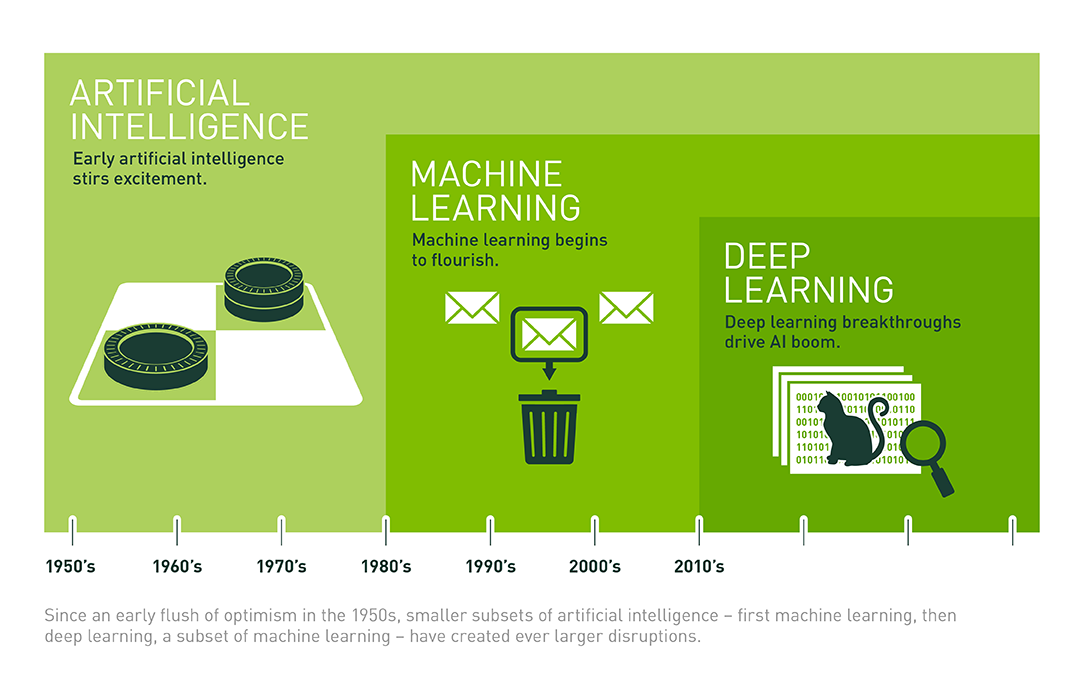## 机器学习的六大步骤

1. 选择数据：将数据集分成三部分，分别是训练集、验证集和测试集。

2. 模型数据：用训练集来构建相关特征的模型。

3. 验证模型：将验证数据导入到模型中。

4. 测试模型：测试集检查被验证模型的表现。

5. 使用模型：训练好的模型在新数据上做预测，分类，聚类。

6. 调优模型：用更多数据、不同的特征或调整过的参数来提升算法的性能表现，提高模型的泛化性。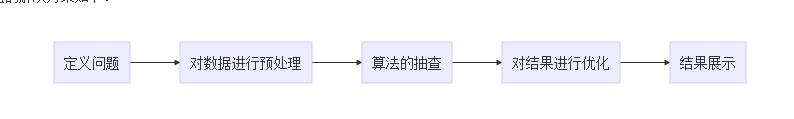• 定义问题

• 对数据进行预处理

• 数据的分析

• 算法的抽查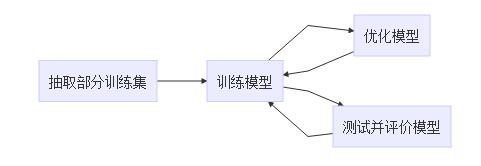• 优化

- 算法调优（Algorithm tuning）

- 集成方法/集成学习（Ensembles）

- 极端特征工程（Extreme Feature Engineering）


### 集成方法/集成学习：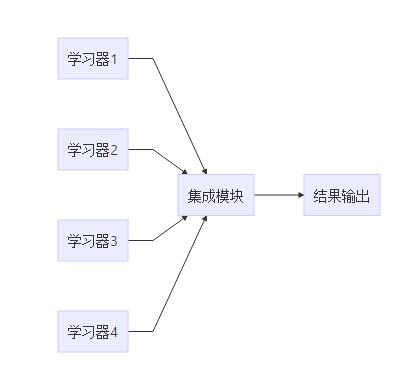Boosting是学习器之间存在强依赖关系。

Bagging与随机森林是学习器之间不存在强依赖关系，相互独立。

### 极端特征工程：

• 结果展示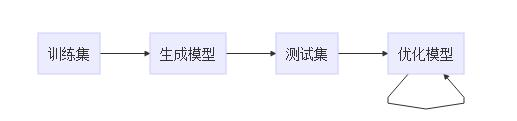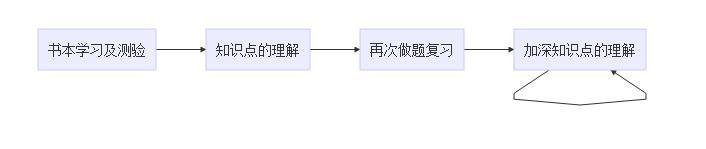# 机器学习的起源与历史

## 人工智能发展史概述

• 1950年以前，统计方法被发现和并不断被改进。

• 1950年代，使用简单的算法进行开创性机器学习研究。

• 1970年代，机器学习表现性能不高的原因，悲观主义盛行，机器学习研究进入寒冬。

• 1980年代，反向传播的重新发现导致机器学习研究的复苏。

• 1990年代，机器学习的工作从知识驱动的方式转变为数据驱动的方法。科学家们开始创建计算机程序来分析大量数据，并从结果中得出结论或“学习”。支持向量机和复现神经网络变得流行起来。

• 2000年代，深度学习重新被广泛使用，神经网络看到广泛的商业用途。

• 2010年代，机器学习成为许多被广泛使用的软件服务的组成部分，并得到各大厂商的大力宣传。纷纷推出自己平台的计算硬件。

## 机器学习大事件纪年表

• 【贝叶斯定理的基础】1763年，托马斯·贝耶斯（ThomasBayes）的作品“解决机会原则”中的一个问题是在他死后两年出版的，经过贝叶斯的朋友理查德·价格的修改和编辑。这篇文章提出了支撑贝叶斯定理的工作。

• 【贝叶斯定理】1812年，Pierre-Simon Laplace发行ThéorieAnalytiquedesProbabilités，他扩展了贝叶斯的作品，并定义了现在称为贝叶斯定理。

• 【马尔可夫链】1913年，安德烈·马可夫首先描述了他用来分析一首诗的技巧。 这种技术后来被称为马尔科夫链。

• 【图灵机】1950年，艾伦·图灵（AlanTuring）提出了一种可以学习和人工智能的“学习机器”。图灵的具体建议预示着遗传算法的出现。

• 【第一个神经网络】1951年，Marvin Minsky和Edmonds建立了第一台能够学习SNARC的神经网络机器。

• 【机器跳棋程序】1952年，Arthur Samuel加入IBM的Poughkeepsie实验室，并开始研究一些机器学习计划，首先开发出下跳棋程序。

• 【感知机】1957年，Frank Rosenblatt在康奈尔航空实验室工作时发明了感知器算法。 大量的媒体报道了感知器算法被发明出来。

• 【k近邻算法（knn）】1967年，创建最近邻算法，这是基本模式识别的开始。 该算法被广泛应用于映射路由。

• 【神经网络的局限性】1969年，Marvin Minsky和Seymour Papert出版了他们的书Perceptrons，描述了感知器和神经网络的一些限制。 这本书显示神经网络基本上受到限制的解释被视为对神经网络研究的障碍。

• 【斯坦福购物车】1979年，斯坦福大学的学生开发了一个可以导航和避开房间障碍物的推车。

• 【神经认知机】1980年，邦彦福岛首先出版他的Neocognitron作品，这是一种人工神经网络。认知后来激发卷积神经网络。

• 【基于解释学习】1981年，Gerald Dejong介绍了基于说明的学习，其中计算机算法分析数据并创建可以遵循的一般规则，并丢弃不重要的数据。

• 【递归神经网络】1982年，约翰·霍普菲尔德（Hop Hopfield）普及了霍普菲尔德网络（Hopfield Networks），一种可用作可内容寻址的内存系统的循环神经网络。

• 【NetTalk】1985年，Terry Sejnowski开发了一种学习用与婴儿相同的方式发音的程序。

• 【反向传播】1986年，反向传播的过程由David Rumelhart，Geoff Hinton和Ronald J. Williams描述。

• 【强化学习】1989年，克里斯托弗·沃特曼斯（Christopher Watkins）开发Q-learning，大大提高了强化学习的实用性和可行性。

• 【个人电脑上机器学习的商业化】1989年，Axcelis公司发布Evolver，这是第一个在个人电脑上使用遗传算法进行商业化的软件包。

• 【玩西洋双陆棋的机器】1992年，杰拉尔德·泰索罗（Gerald Tesauro）开发了TD-Gammon，一款计算机西洋双陆棋程序，它利用了使用时差学习训练的人造神经网络（因此以“TD”的名义）。 TD-Gammon能够与顶级人类西洋双陆棋玩家的能力相提并论，但并不总是超越。

• 【随机森林算法】1995年，田锦浩出版了一篇描述随机决策林的论文。

• 【IBM深蓝击败卡斯帕罗夫】1997年，IBM的Deep Blue在国际象棋中击败世界冠军。

• 【LSTM】1997年，Sepp Hochreiter和JürgenSchmidhuber发明了长期记忆复发神经网络，大大提高了复发神经网络的效率和实用性。

• 【MNIST数据库】1998年，由Yann LeCun领导的团队发布了MNIST数据库，这是一个数据集，其中包含美国人口普查局员工和美国高中学生的手写数字。MNIST数据库已成为评估手写识别的基准。

• 【火炬机器学习库】2002年，火炬机器学习库首次发布。

• 【Netflix奖】2006年，Netflix大奖由Netflix推出。 比赛的目的是使用机器学习来击败Netflix自己的推荐软件的准确性，以预测用户对以前电影的评分至少10％的电影评级。该奖项在2009年获胜。

• 【Kaggle比赛】2010年，Kaggle是一个作为机器学习竞赛平台的网站。

• 【打败人类】2011年，使用机器学习，自然语言处理和信息检索技术的组合，IBM的沃森击败了两个人类的冠军。

# 机器学习的几个重要概念

## 奥卡姆剃刀

“若有多个假设与观察一致，则选择最简单的那一个。”即在多个模型效果相同的情况下，选择模型最简单的那一个。

## 欠拟合与过拟合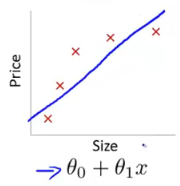### 1. 欠拟合

1. 适当加入特征量。
比如，模型y=x欠拟合，我们可以加入别的特征向量，把模型变成y=x1+x2+x3，这样特征量变多了可以更好的表示模型。

2. 降低正则化参数，正则化的作用是防止过拟合，如果模型出现了欠拟合，则需要降低正则化参数。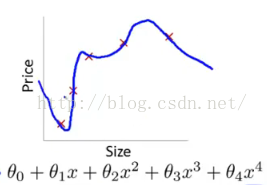### 2. 过拟合

1. 再次清洗数据，数据不纯可能导致结果过拟合。

2. 增加训练集，训练集过小可能导致结果过拟合。

3. 正则化。

## 偏差与方差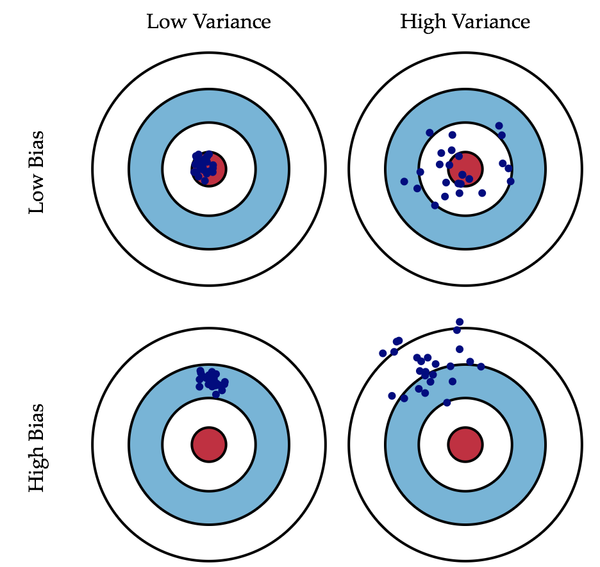# 机器学习常用开发工具

## scikit-learn

http://scikit-learn.org/stable/

## OpenCV(图像处理与毕业论文大杀器)

http://opencv.org/

OpenCV具有C ++，C，Python和Java接口，并支持Windows，Linux，Mac OS，iOS和Android。
OpenCV专为计算效率而设计，并强调实时应用。

OpenCV已经通过世界各地的用户社区超过4.7万人，估计下载量超过1400万。

http://blog.csdn.net/sileixinhua/article/details/72810858

## tensorflow

https://www.tensorflow.org/

TensorFlow™是使用数据流图进行数值计算的开源软件库。

TensorFlow用于进行机器学习和深层神经网络研究，但该系统普遍足以适用于各种其他领域。

## caffe

http://caffe.berkeleyvision.org/

Caffe是一个深度学习框架，特点为速度和模块化。

http://demo.caffe.berkeleyvision.org/
cffe官方一个分类器的demo，可以上传照片然后查看分类结果

## pyTouch

http://pytorch.org/

PyTorch是一个深度学习框架，将Python放在首位。

## Java-ML

http://java-ml.sourceforge.net/

Java-ML简而言之，机器学习算法的集合，有每种算法的公共接口和类型，主要针对软件工程师和程序员，所以没有GUI，但界面清晰，良好的源代码。，大量的代码示例和教程。

## Spark

http://spark.apache.org/

Apache Spark是用于大规模数据处理的快速和通用引擎。

## NumPy,pandas,matplotlib

NumPy是使用Python进行科学计算的基础软件包。

pandas为Python编程语言提供高性能，易于使用的数据结构和数据分析工具。

Matplotlib是一个Python 2D绘图库，可以跨平台生成各种硬拷贝格式和交互式环境的出版品质量图。 Matplotlib可用于Python脚本，Python和IPython shell，jupyter笔记本，Web应用程序服务器和四个图形用户界面工具包。

## keras

Keras是一种高级神经网络API，用Python编写，能够运行在TensorFlow，CNTK或Theano之上。

## theano

http://deeplearning.net/software/theano/

Theano是一个Python库，定义，优化和评估涉及多维数组的数学表达式。

## NLTK

http://www.nltk.org/

NLTK是构建Python程序以处理人类语言数据的领先平台。

## jieba

https://pypi.python.org/pypi/jieba/

“结巴”中文分词：做最好的 Python 中文分词组件。

## Storm

http://storm.apache.org/

## Julia/R

https://julialang.org/

https://www.r-project.org/

Julia是数字计算中高性能动态编程语言。

Julia的基础库主要用于Julia本身，还集成了成熟的，最好的开源C和Fortran库，用于线性代数，随机数生成，信号处理和字符串处理。

Jupyter和Julia社区合作的IJulia为Julia提供了强大的基于浏览器的图形笔记本界面。

R是用于统计计算和图形的免费软件环境。 它可以在各种UNIX平台（Windows和MacOS）上编译和运行。

## Weka

http://www.cs.waikato.ac.nz/ml/weka/

Weka是数据挖掘任务的机器学习算法的集合。

Weka包含用于数据预处理，分类，回归，聚类，关联规则和可视化的工具。

# 机器学习初步实战

## 数据分析入门：Weka的使用（只要四步，不会编码也可以做机器学习与数据分析）

2. 点击Explorer。
3. 点击Open file…选择weka安装文件夹下的data文件夹中的iris.arff数据文件。
4. 点击Classify->choose,选择bayes下的nativeBayes算法，让后点击start。显示以下结果。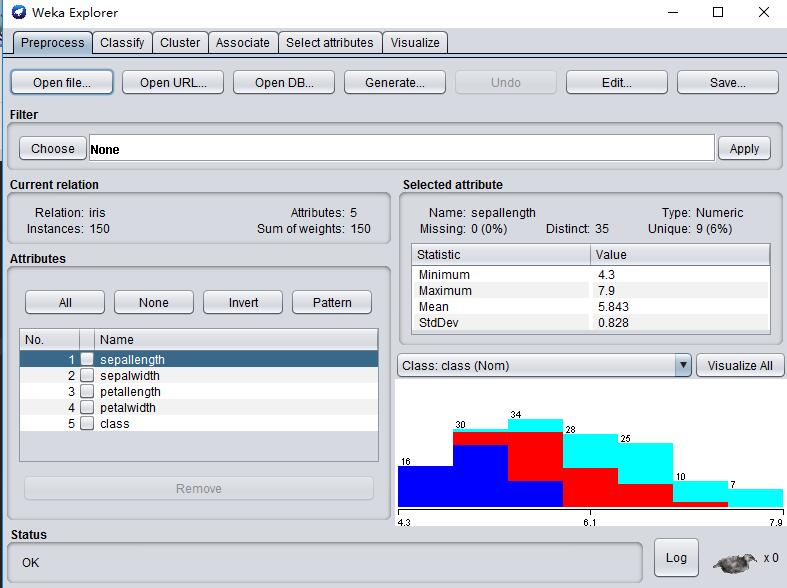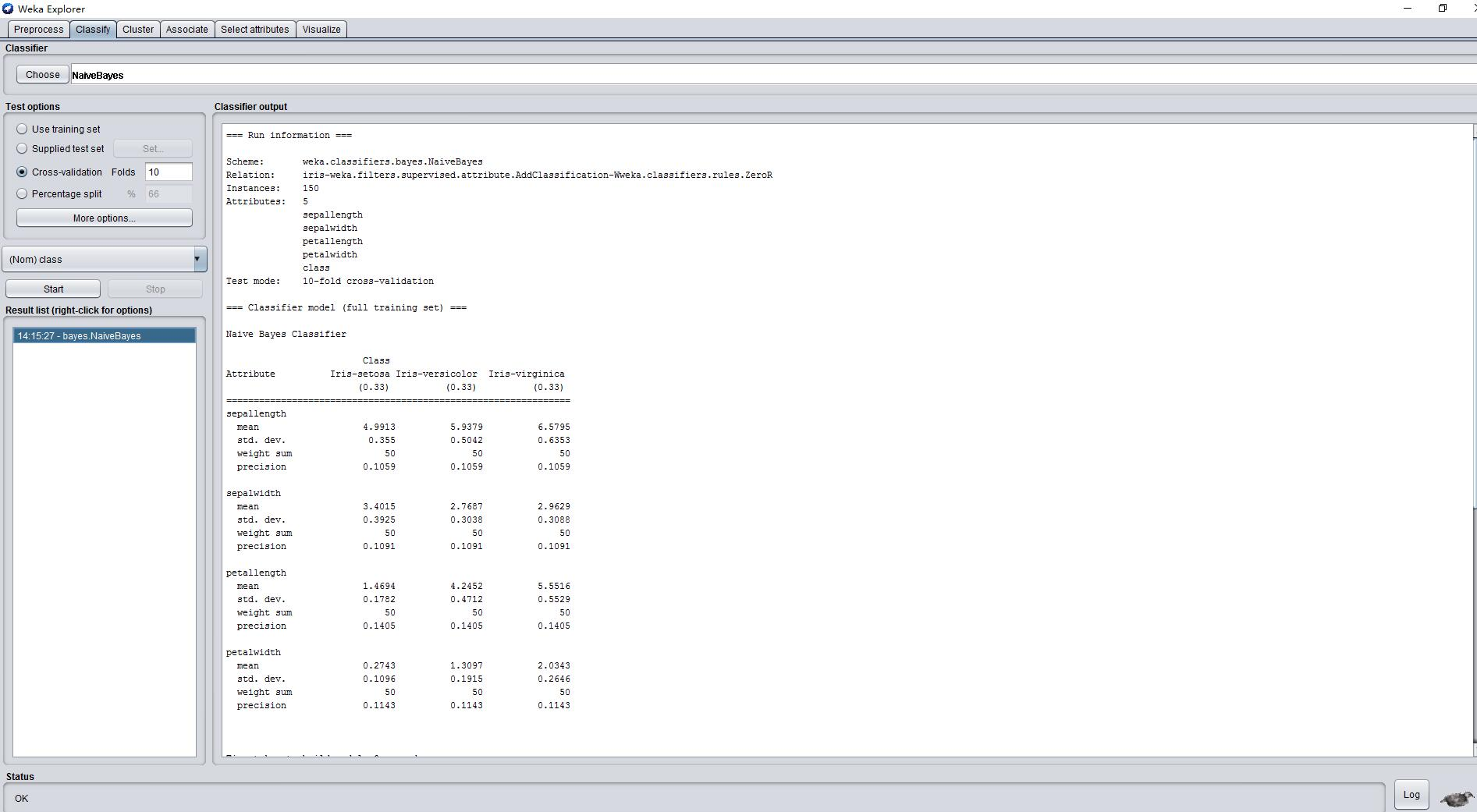=== Run information ===

Scheme:       weka.classifiers.bayes.NaiveBayes
Instances:    150
Attributes:   5
sepallength
sepalwidth
petallength
petalwidth
class
Test mode:    10-fold cross-validation

=== Classifier model (full training set) ===

Naive Bayes Classifier

Class
Attribute          Iris-setosa Iris-versicolor  Iris-virginica
(0.33)          (0.33)          (0.33)
===============================================================
sepallength
mean                   4.9913          5.9379          6.5795
std. dev.               0.355          0.5042          0.6353
weight sum                 50              50              50
precision              0.1059          0.1059          0.1059

sepalwidth
mean                   3.4015          2.7687          2.9629
std. dev.              0.3925          0.3038          0.3088
weight sum                 50              50              50
precision              0.1091          0.1091          0.1091

petallength
mean                   1.4694          4.2452          5.5516
std. dev.              0.1782          0.4712          0.5529
weight sum                 50              50              50
precision              0.1405          0.1405          0.1405

petalwidth
mean                   0.2743          1.3097          2.0343
std. dev.              0.1096          0.1915          0.2646
weight sum                 50              50              50
precision              0.1143          0.1143          0.1143

Time taken to build model: 0 seconds

=== Stratified cross-validation ===
=== Summary ===

Correctly Classified Instances         144               96      %
Incorrectly Classified Instances         6                4      %
Kappa statistic                          0.94
Mean absolute error                      0.0342
Root mean squared error                  0.155
Relative absolute error                  7.6997 %
Root relative squared error             32.8794 %
Total Number of Instances              150

=== Detailed Accuracy By Class ===

TP Rate  FP Rate  Precision  Recall   F-Measure  MCC      ROC Area  PRC Area  Class
1.000    0.000    1.000      1.000    1.000      1.000    1.000     1.000     Iris-setosa
0.960    0.040    0.923      0.960    0.941      0.911    0.992     0.983     Iris-versicolor
0.920    0.020    0.958      0.920    0.939      0.910    0.992     0.986     Iris-virginica
Weighted Avg.    0.960    0.020    0.960      0.960    0.960      0.940    0.994     0.989

=== Confusion Matrix ===

a  b  c   <-- classified as
50  0  0 |  a = Iris-setosa
0 48  2 |  b = Iris-versicolor
0  4 46 |  c = Iris-virginica



## 机器学习的“Hello World” MNIST手写字体识别（用SVM支持向量机算法）

print(__doc__)

import matplotlib.pyplot as plt
# 载入matplotlib中画图库
from sklearn import datasets, svm, metrics
# 载入sklearn中样本数据集，svm算法，和矩阵处理库

# 导入datasets样本数据集中 MNIST手写字体识别数据进digits

images_and_labels = list(zip(digits.images, digits.target))
# 导入的数据分类图像和标签两部分，即数字图像和对应的数字标签
for index, (image, label) in enumerate(images_and_labels[:4]):
plt.subplot(2, 4, index + 1)
plt.axis('off')
plt.imshow(image, cmap=plt.cm.gray_r, interpolation='nearest')
plt.title('Training: %i' % label)
# 包括标签和图像在内的一共8组训练图像

n_samples = len(digits.images)
# 获取样本数
data = digits.images.reshape((n_samples, -1))
# 将图像转换成矩阵

classifier = svm.SVC(gamma=0.001)
# 使用SVM算法

classifier.fit(data[:n_samples // 2], digits.target[:n_samples // 2])
# 分类图像

expected = digits.target[n_samples // 2:]
predicted = classifier.predict(data[n_samples // 2:])
# 计算预测值

print("Classification report for classifier %s:\n%s\n"
% (classifier, metrics.classification_report(expected, predicted)))
# 输出分类后的结果信息
print("Confusion matrix:\n%s" % metrics.confusion_matrix(expected, predicted))
# 输出混淆矩阵（confusion_matrix）下面介绍什么是混淆矩阵

images_and_predictions = list(zip(digits.images[n_samples // 2:], predicted))
for index, (image, prediction) in enumerate(images_and_predictions[:4]):
plt.subplot(2, 4, index + 5)
plt.axis('off')
plt.imshow(image, cmap=plt.cm.gray_r, interpolation='nearest')
plt.title('Prediction: %i' % prediction)
# 包括标签和图像在内的一共8组预测图像

plt.show()
# 输出结果图像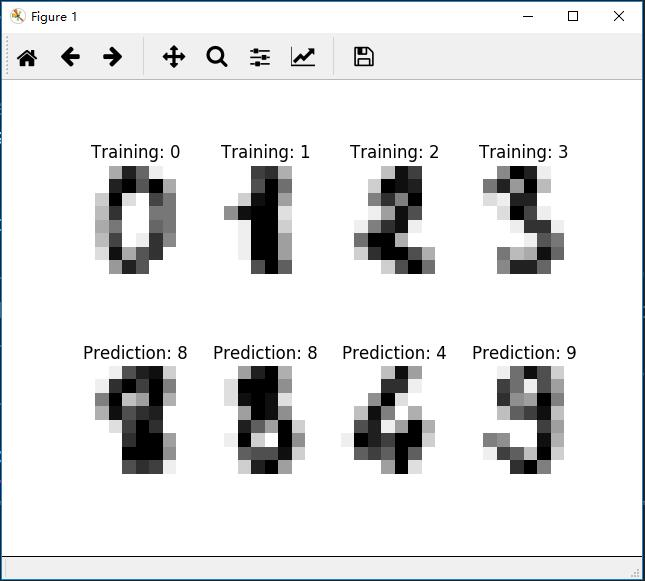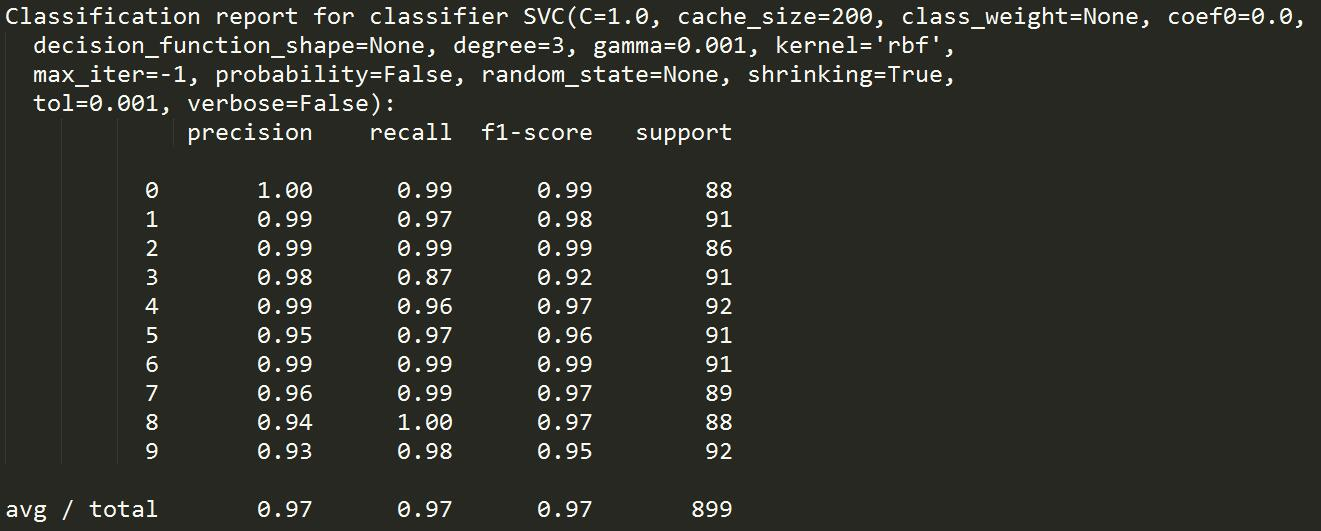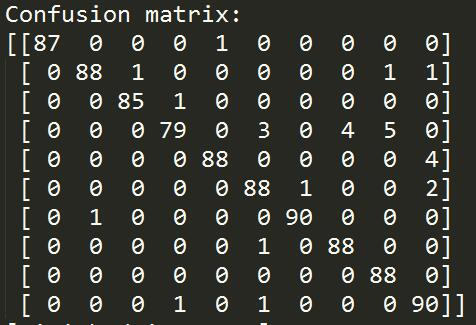# 混淆矩阵（confusion_matrix）

option Negative Positive
Negative A B
Positive C D

- a 表示伪的预测正确值 即错误的信息被预测为错误的信息 预测正确
- b 表示伪的预测错误值 即错误的信息被预测为正确的信息 预测错误
- c 表示真的预测错误值 即错误的正确被预测为错误的信息 预测正确
- d 表示真的预测正确值 即错误的正确被预测为正确的信息 预测错误

## Iris花数据集分析

print(__doc__)

import matplotlib.pyplot as plt
# 导入matplotlib画图
from mpl_toolkits.mplot3d import Axes3D
# 导入mpl_toolkits画3D图像
from sklearn import datasets
# 导入sklearn自带的训练集
from sklearn.decomposition import PCA
# 导入特征降维的PCA主成分分析法

# 导入iris花数据集进iris变量中
X = iris.data[:, :2]
# 导入图像数据给X变量，只使用头两个特征向量
y = iris.target
# 导入图像标签给Y，即图像的结果，如1.2.3...9

x_min, x_max = X[:, 0].min() - .5, X[:, 0].max() + .5
y_min, y_max = X[:, 1].min() - .5, X[:, 1].max() + .5
# 设置下，y的最大值和最小值

plt.figure(2, figsize=(8, 6))
plt.clf()

plt.scatter(X[:, 0], X[:, 1], c=y, cmap=plt.cm.Paired)
# 设置输出图像为散点图
plt.xlabel('Sepal length')
plt.ylabel('Sepal width')
# 设置输出图像的X轴和Y轴的标签

plt.xlim(x_min, x_max)
plt.ylim(y_min, y_max)
plt.xticks(())
plt.yticks(())

fig = plt.figure(1, figsize=(8, 6))
ax = Axes3D(fig, elev=-150, azim=110)
# 设置3D图像
X_reduced = PCA(n_components=3).fit_transform(iris.data)
#用PCA给特征向量降维
ax.scatter(X_reduced[:, 0], X_reduced[:, 1], X_reduced[:, 2], c=y,
cmap=plt.cm.Paired)
# 在3D图像中显示散点信息
ax.set_title("First three PCA directions")
# 设置3D图像标题
ax.set_xlabel("1st eigenvector")
ax.w_xaxis.set_ticklabels([])
ax.set_ylabel("2nd eigenvector")
ax.w_yaxis.set_ticklabels([])
ax.set_zlabel("3rd eigenvector")
ax.w_zaxis.set_ticklabels([])
# 设置3D图像的X,Y,Z轴

plt.show()
# 输出图像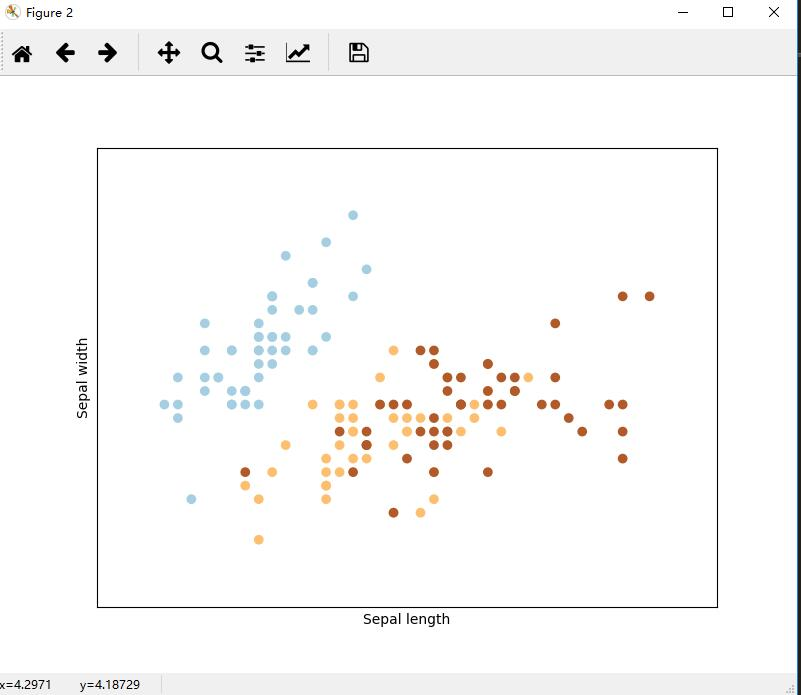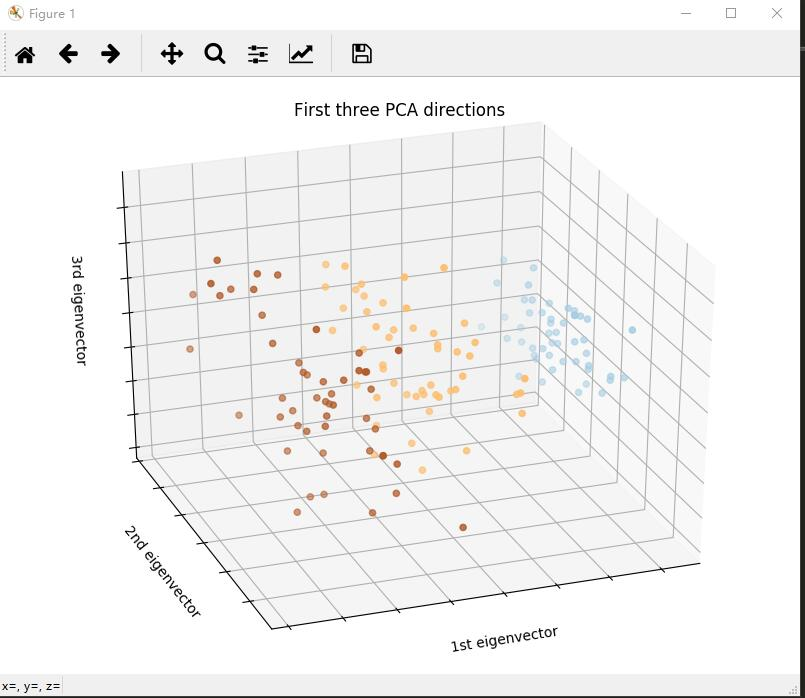## 支持向量机（SVM）人脸识别

from __future__ import print_function

from time import time
import logging
import matplotlib.pyplot as plt

from sklearn.model_selection import train_test_split
from sklearn.model_selection import GridSearchCV
from sklearn.datasets import fetch_lfw_people
from sklearn.metrics import classification_report
from sklearn.metrics import confusion_matrix
from sklearn.decomposition import PCA
from sklearn.svm import SVC
# 导入必要的数据集和算法

print(__doc__)

# 在stdout上显示进度日志
logging.basicConfig(level=logging.INFO, format='%(asctime)s %(message)s')

lfw_people = fetch_lfw_people(min_faces_per_person=70, resize=0.4)

# 图像数组以找到形状（绘图）
n_samples, h, w = lfw_people.images.shape

# 对于机器学习，我们直接使用2个数据（由于该模型忽略了相对像素位置信息）
X = lfw_people.data
n_features = X.shape

# 预测的标签是该人的身份
y = lfw_people.target
# y为特征脸的标签
target_names = lfw_people.target_names
# 设置标签的名字
n_classes = target_names.shape

print("Total dataset size:")
print("n_samples: %d" % n_samples)
print("n_features: %d" % n_features)
print("n_classes: %d" % n_classes)

# 分为测试集和测试集
X_train, X_test, y_train, y_test = train_test_split(
X, y, test_size=0.25, random_state=42)
# 测试集大小为全部数据集的25%

n_components = 150

print("Extracting the top %d eigenfaces from %d faces"
% (n_components, X_train.shape))
t0 = time()
# 记时
pca = PCA(n_components=n_components, svd_solver='randomized',
whiten=True).fit(X_train)
# 设置PCA降维
print("done in %0.3fs" % (time() - t0))
# 输出总耗时

eigenfaces = pca.components_.reshape((n_components, h, w))
# 将图像转换为矩阵向量

print("Projecting the input data on the eigenfaces orthonormal basis")
t0 = time()
X_train_pca = pca.transform(X_train)
# 在测试集上PCA降维
X_test_pca = pca.transform(X_test)
# 在数据集上PCA降维
print("done in %0.3fs" % (time() - t0))

print("Fitting the classifier to the training set")
t0 = time()
param_grid = {'C': [1e3, 5e3, 1e4, 5e4, 1e5],
'gamma': [0.0001, 0.0005, 0.001, 0.005, 0.01, 0.1], }
clf = GridSearchCV(SVC(kernel='rbf', class_weight='balanced'), param_grid)
clf = clf.fit(X_train_pca, y_train)
print("done in %0.3fs" % (time() - t0))
print("Best estimator found by grid search:")
print(clf.best_estimator_)

print("Predicting people's names on the test set")
t0 = time()
y_pred = clf.predict(X_test_pca)
print("done in %0.3fs" % (time() - t0))

print(classification_report(y_test, y_pred, target_names=target_names))
print(confusion_matrix(y_test, y_pred, labels=range(n_classes)))

def plot_gallery(images, titles, h, w, n_row=3, n_col=4):
"""Helper function to plot a gallery of portraits"""
plt.figure(figsize=(1.8 * n_col, 2.4 * n_row))
for i in range(n_row * n_col):
plt.subplot(n_row, n_col, i + 1)
plt.imshow(images[i].reshape((h, w)), cmap=plt.cm.gray)
plt.title(titles[i], size=12)
plt.xticks(())
plt.yticks(())

# 绘制测试结果的一部分

def title(y_pred, y_test, target_names, i):
pred_name = target_names[y_pred[i]].rsplit(' ', 1)[-1]
true_name = target_names[y_test[i]].rsplit(' ', 1)[-1]
return 'predicted: %s\ntrue:      %s' % (pred_name, true_name)

prediction_titles = [title(y_pred, y_test, target_names, i)
for i in range(y_pred.shape)]

plot_gallery(X_test, prediction_titles, h, w)

# 绘制特征脸

eigenface_titles = ["eigenface %d" % i for i in range(eigenfaces.shape)]
plot_gallery(eigenfaces, eigenface_titles, h, w)

plt.show()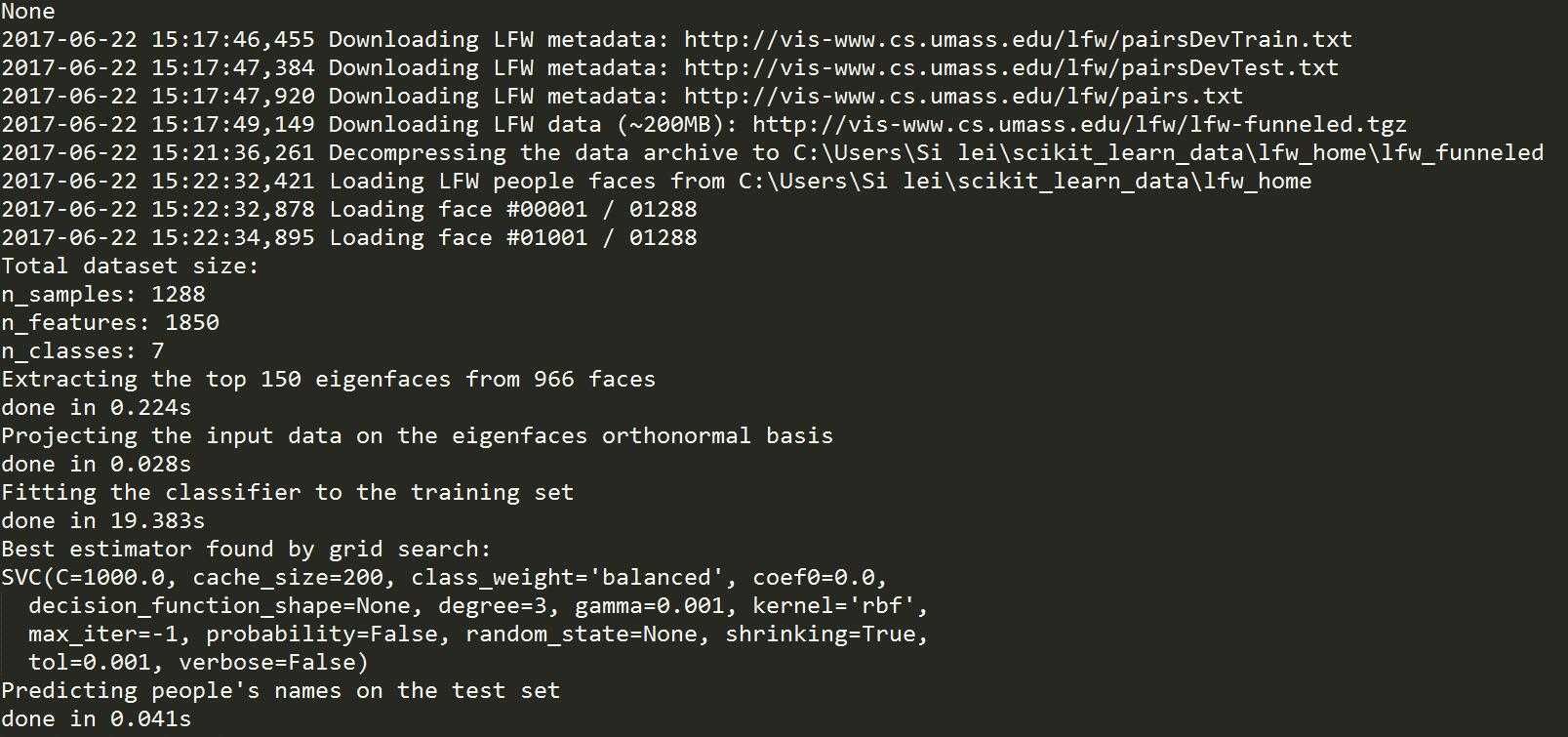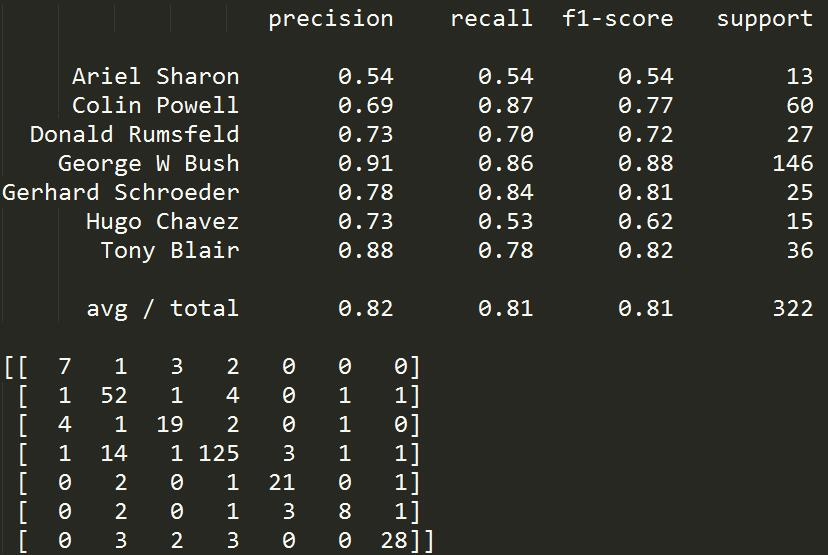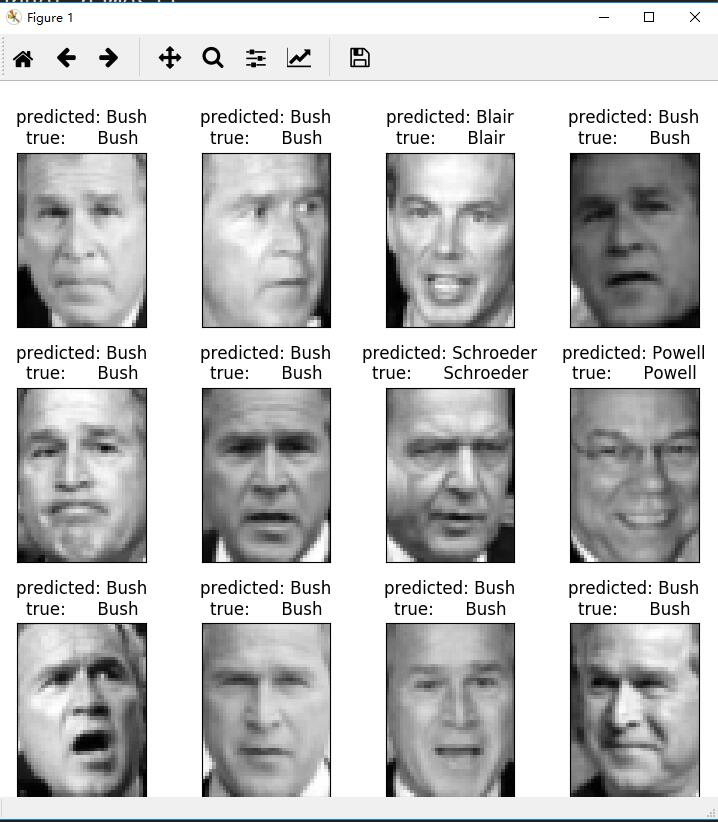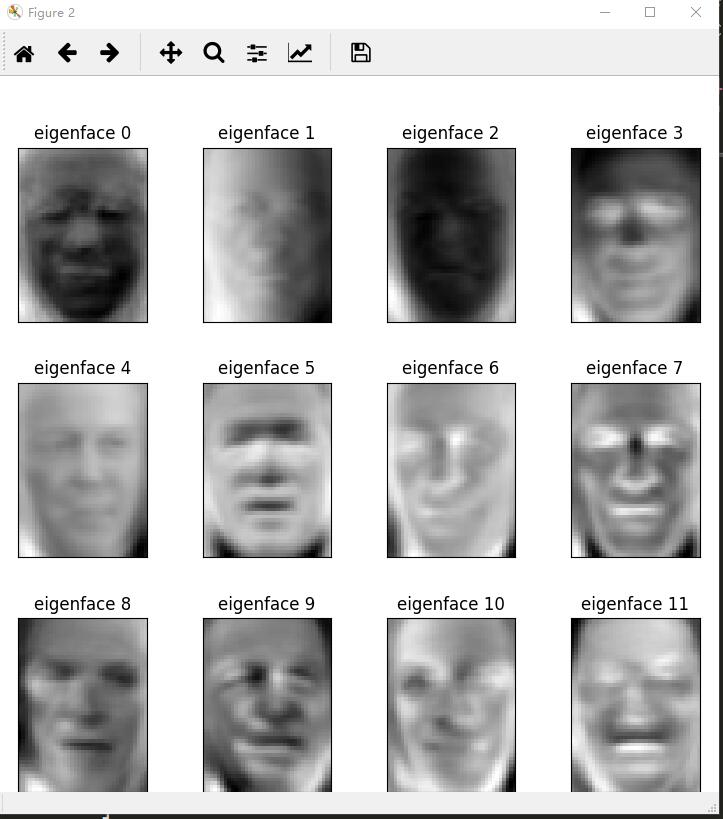## 多层感知机识别MNIST手写字体识

1.安装tensorlfow

python.exe -m pip install --upgrade pip
pip install tensorflow

2.复制粘贴并运行以下代码,下载数据集要花一段时间

import tensorflow as tf
# 导入tensorflow

def weight_variable(shape):
initial = tf.truncated_normal(shape, stddev=0.1)
return tf.Variable(initial)
# 这里可以初步理解为k
# 我们设立一个y=kx+b的方程，我们导入数据x，y，有很多个这样二元一次方程被设立
# 根据这个二元一次方程组可以求出k和b的值，然后带入新的数据x，则可以求出结果y

def bias_variable(shape):
initial = tf.constant(0.1, shape=shape)
return tf.Variable(initial)
# 同理这里可以初步理解为b

def conv2d(x, W):
# 转换为2d

def max_pool_2x2(x):
# 设置最大为2x2

sess = tf.InteractiveSession()
# 设置sess

x = tf.placeholder("float", shape=[None, 784])
# 设置X输入数组
x_image = tf.reshape(x, [-1,28,28,1])
W_conv1 = weight_variable([5,5,1,32])
b_conv1 = bias_variable()
h_conv1 = tf.nn.relu(conv2d(x_image, W_conv1) + b_conv1)
h_pool1 = max_pool_2x2(h_conv1)
W_conv2 = weight_variable([5,5,32,64])
b_conv2 = bias_variable()
h_conv2 = tf.nn.relu(conv2d(h_pool1,W_conv2) + b_conv2)
h_pool2 = max_pool_2x2(h_conv2)
W_fc1 = weight_variable([7*7*64,1024])
b_fc1 = bias_variable()
h_pool2_flat = tf.reshape(h_pool2, [-1, 7*7*64])
h_fc1 = tf.nn.relu(tf.matmul(h_pool2_flat, W_fc1) + b_fc1)
keep_prob = tf.placeholder("float")
h_fc1_drop = tf.nn.dropout(h_fc1, keep_prob)
W_fc2 = weight_variable([1024,10])
b_fc2 = bias_variable()
y_conv = tf.nn.softmax(tf.matmul(h_fc1_drop, W_fc2) + b_fc2)
# 设置激活函数
y_ = tf.placeholder("float", shape=[None, 10])
# 设置结果y
cross_entropy = -tf.reduce_sum(y_*tf.log(y_conv))
# 设置训练步长
correct_prediction = tf.equal(tf.argmax(y_conv,1), tf.argmax(y_,1))
# 设置正确预测
accuracy = tf.reduce_mean(tf.cast(correct_prediction, "float"))
# 设置结果精确度

sess.run(tf.initialize_all_variables())
# 运行

from tensorflow.examples.tutorials.mnist import input_data

for i in range(20000):
batch = mnist.train.next_batch(50)
if i % 100 == 0:
feed_dict = {x:batch,y_:batch,keep_prob:1.0}
train_accuracy = accuracy.eval(feed_dict=feed_dict)
print("step %d, training accuracy %g" % (i, train_accuracy))
train_step.run(feed_dict={x:batch,y_:batch,keep_prob:0.5})
#不断训练模型，根新参数

feed_dict={x:mnist.test.images, y_: mnist.test.labels,keep_prob:1.0}
print("test accuracy %g" % accuracy.eval(feed_dict=feed_dict))
# 输出结果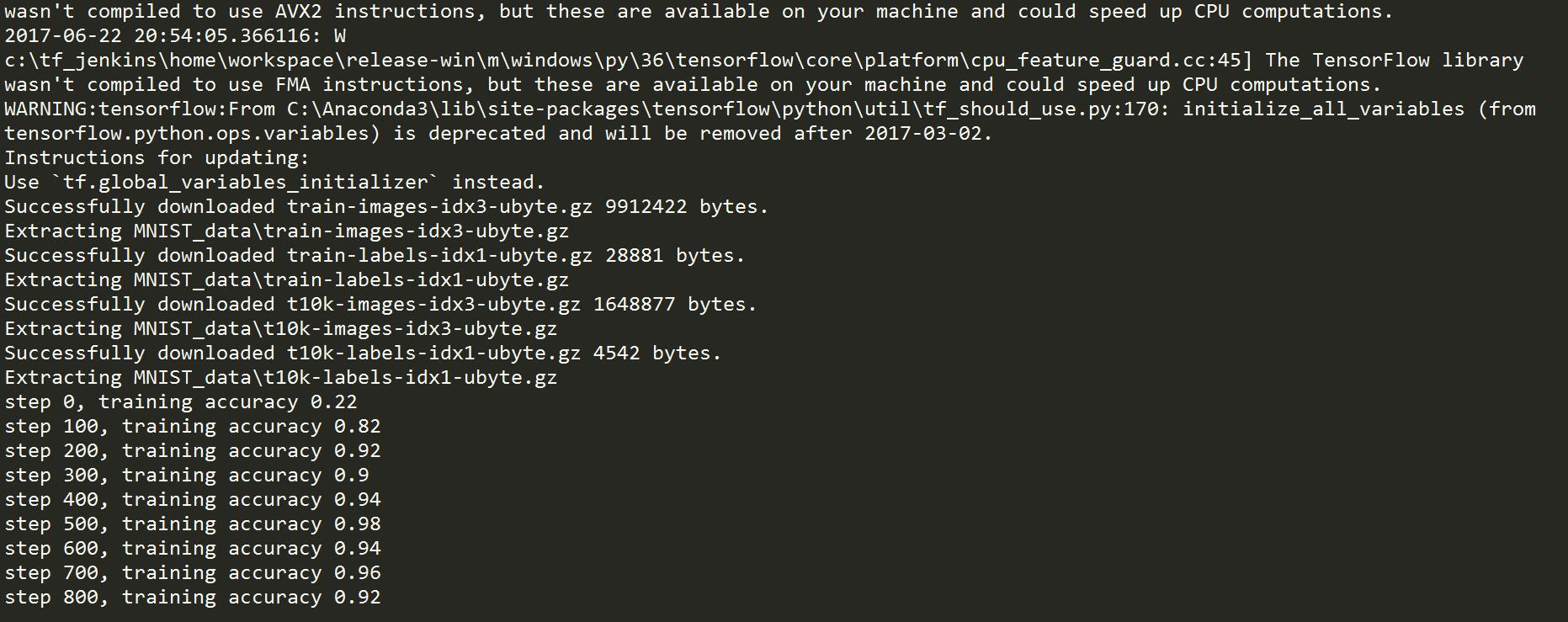# 参考文献

## 更新列表

#### 2017年6月23日15:50:42

1. 加上机器学习和深度学习的区别
2. 加上weka这个工具的介绍
3. 实战段落修改
4. 什么是机器学习那一段，再放前面来
5. 机器学习的起源和历史，放到概述后面

——————————————————————————————————-

QQ群号： 657119450